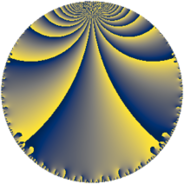# Properties

 Label 147.6.mLevel $147$ Weight $6$ Character orbit 147.m Rep. character $\chi_{147}(4,\cdot)$ Character field $\Q(\zeta_{21})$ Dimension $564$ Sturm bound $112$

# Related objects

## Defining parameters

 Level: $$N$$ $$=$$ $$147 = 3 \cdot 7^{2}$$ Weight: $$k$$ $$=$$ $$6$$ Character orbit: $$[\chi]$$ $$=$$ 147.m (of order $$21$$ and degree $$12$$) Character conductor: $$\operatorname{cond}(\chi)$$ $$=$$ $$49$$ Character field: $$\Q(\zeta_{21})$$ Sturm bound: $$112$$

## Dimensions

The following table gives the dimensions of various subspaces of $$M_{6}(147, [\chi])$$.

Total New Old
Modular forms 1140 564 576
Cusp forms 1092 564 528
Eisenstein series 48 0 48

## Trace form

 $$564 q + 9 q^{3} + 768 q^{4} - 22 q^{5} - 144 q^{6} - 167 q^{7} + 492 q^{8} + 3807 q^{9} + O(q^{10})$$ $$564 q + 9 q^{3} + 768 q^{4} - 22 q^{5} - 144 q^{6} - 167 q^{7} + 492 q^{8} + 3807 q^{9} + 1182 q^{10} + 1244 q^{11} + 288 q^{12} - 2158 q^{13} - 4558 q^{14} + 990 q^{15} + 13560 q^{16} - 826 q^{17} - 17559 q^{19} - 11304 q^{20} + 4068 q^{21} - 3300 q^{22} + 2552 q^{23} + 3024 q^{24} + 26357 q^{25} - 10870 q^{26} - 1458 q^{27} + 38380 q^{28} + 992 q^{29} - 1368 q^{30} - 79999 q^{31} - 55466 q^{32} + 11430 q^{33} + 74760 q^{34} - 1066 q^{35} - 124416 q^{36} - 12788 q^{37} - 45286 q^{38} - 106416 q^{39} - 58114 q^{40} - 58108 q^{41} - 66312 q^{42} - 10514 q^{43} - 16128 q^{44} + 23166 q^{45} + 20180 q^{46} + 195304 q^{47} + 65808 q^{48} + 163043 q^{49} - 487348 q^{50} + 67860 q^{51} - 29172 q^{52} - 54400 q^{53} + 5832 q^{54} - 137012 q^{55} - 672144 q^{56} + 24354 q^{57} - 225162 q^{58} - 318496 q^{59} - 79362 q^{60} - 58417 q^{61} + 508444 q^{62} + 60264 q^{63} - 86288 q^{64} + 75342 q^{65} + 2988 q^{66} - 13069 q^{67} - 66612 q^{68} - 154224 q^{69} + 27876 q^{70} + 398388 q^{71} - 159408 q^{72} - 78511 q^{73} - 502714 q^{74} + 48807 q^{75} - 251626 q^{76} + 171826 q^{77} + 90468 q^{78} - 35877 q^{79} + 765886 q^{80} + 308367 q^{81} + 725358 q^{82} - 15286 q^{83} - 47160 q^{84} + 231816 q^{85} - 923244 q^{86} - 388674 q^{87} - 1168704 q^{88} - 615110 q^{89} - 191484 q^{90} - 443123 q^{91} - 316932 q^{92} - 120717 q^{93} + 1690084 q^{94} + 1531074 q^{95} + 164790 q^{96} + 3116244 q^{97} + 2271326 q^{98} - 22356 q^{99} + O(q^{100})$$

## Decomposition of $$S_{6}^{\mathrm{new}}(147, [\chi])$$ into newform subspaces

The newforms in this space have not yet been added to the LMFDB.

## Decomposition of $$S_{6}^{\mathrm{old}}(147, [\chi])$$ into lower level spaces

$$S_{6}^{\mathrm{old}}(147, [\chi]) \cong$$ $$S_{6}^{\mathrm{new}}(49, [\chi])$$$$^{\oplus 2}$$